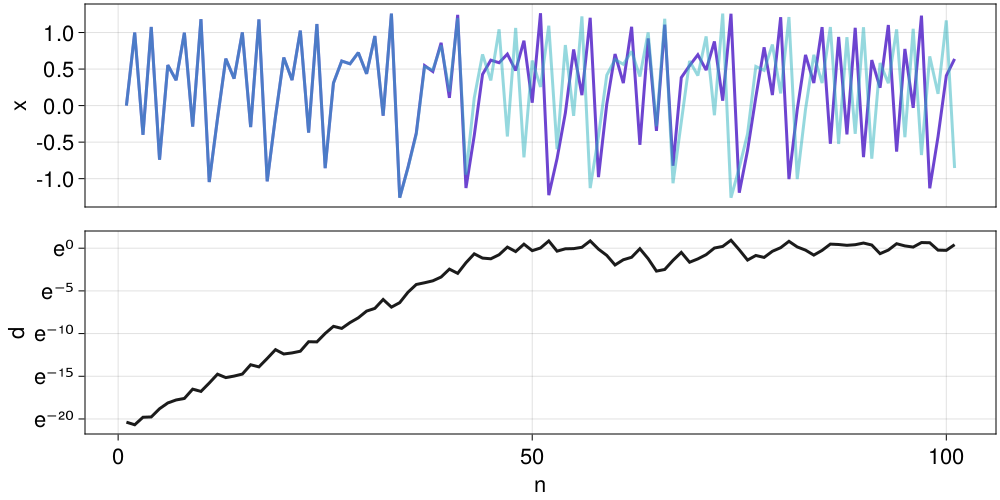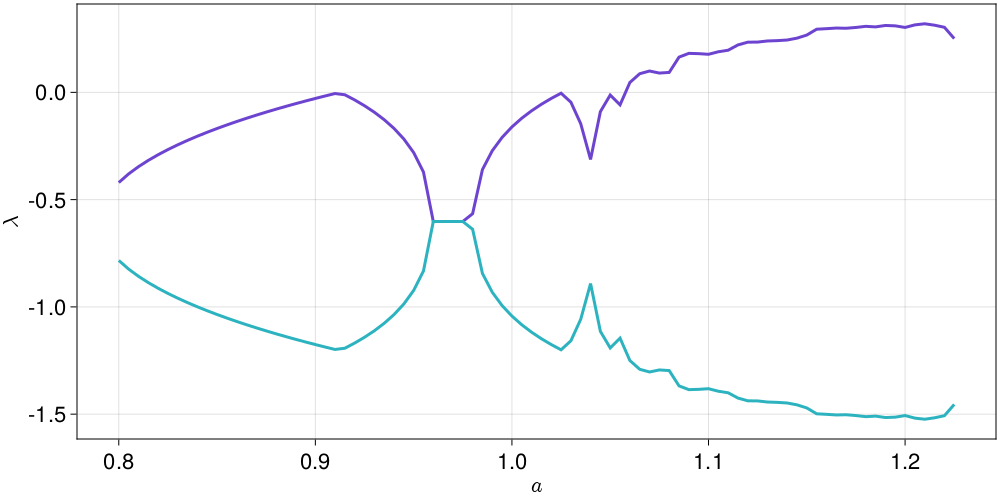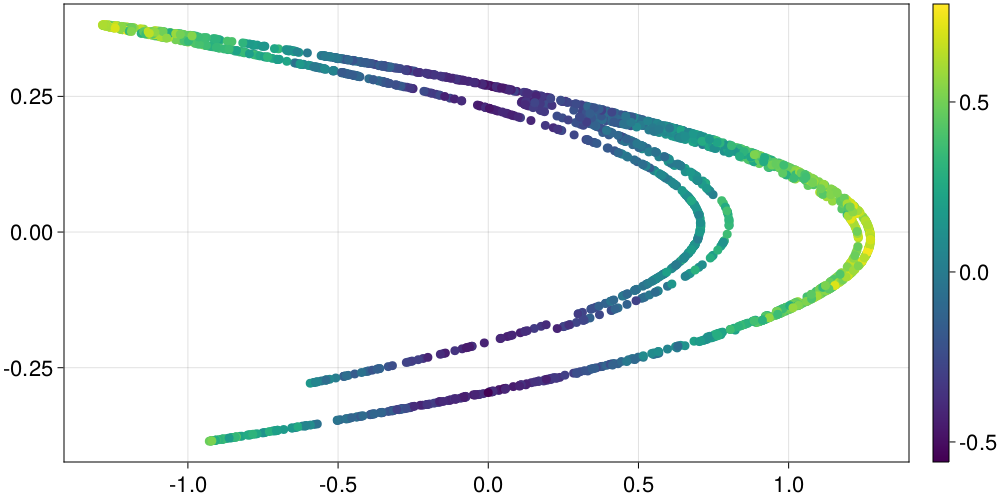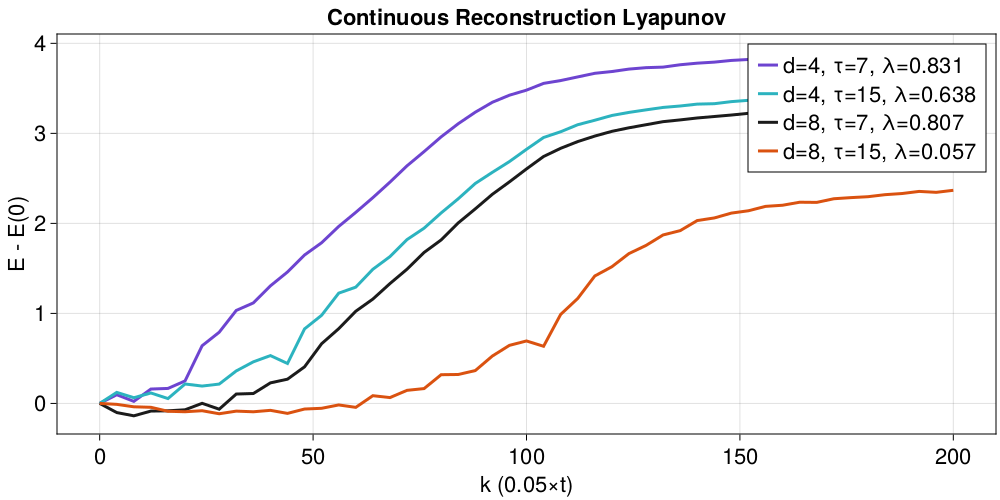# Lyapunov Exponents

Lyapunov exponents measure exponential rates of separation of nearby trajectories in the flow of a dynamical system. The Wikipedia and the Scholarpedia entries have a lot of valuable information about the history and usage of these quantities.

Performance depends on the solver

Notice that the performance of functions that use ContinuousDynamicalSystems depend crucially on the chosen solver. Please see the documentation page on Choosing a solver for an in-depth discussion.

## Concept of the Lyapunov exponent

Before providing the documentation of the offered functionality, it is good to demonstrate exactly what are the Lyapunov exponents.

For chaotic systems, nearby trajectories separate in time exponentially fast (while for stable systems they come close exponentially fast). This happens at least for small separations, and is demonstrated in the following sketch:.

In this sketch $\lambda$ is the maximum Lyapunov exponent (and in general a system has as many exponents as its dimensionality).

Let's demonstrate these concepts using a real system, the Hénon map:

\begin{aligned} x_{n+1} &= 1 - ax_n^2 + y_n \\ y_{n+1} &= bx_n \end{aligned}

Let's get a trajectory

using DynamicalSystems, CairoMakie
henon = Systems.henon()
tr1 = trajectory(henon, 100)
summary(tr1)
"2-dimensional Dataset{Float64} with 101 points"

and create one more trajectory that starts very close to the first one

u2 = get_state(henon) + (1e-9 * ones(dimension(henon)))
tr2 = trajectory(henon, 100, u2)
summary(tr2)
"2-dimensional Dataset{Float64} with 101 points"

We now want to demonstrate how the distance between these two trajectories increases with time:

using LinearAlgebra: norm

fig = Figure()

# Plot the x-coordinate of the two trajectories:
ax1 = Axis(fig[1,1]; ylabel = "x")
lines!(ax1, tr1[:, 1]; color = Cycled(1))
lines!(ax1, tr2[:, 1]; color = (Main.COLORS, 0.5))
hidexdecorations!(ax1; grid = false)

# Plot their distance in a semilog plot:
ax2 = Axis(fig[2,1]; ylabel = "d", xlabel = "n", yscale = log)
d = [norm(tr1[i] - tr2[i]) for i in 1:length(tr2)]
lines!(ax2, d; color = Cycled(3))
figThe initial slope of the d vs n plot (before the curve saturates) is approximately the maximum Lyapunov exponent!

## Lyapunov Spectrum

The function lyapunovspectrum calculates the entire spectrum of the Lyapunov exponents of a system:

ChaosTools.lyapunovspectrumFunction
lyapunovspectrum(ds::DynamicalSystem, N [, k::Int | Q0]; kwargs...) -> λs

Calculate the spectrum of Lyapunov exponents [Lyapunov1992] of ds by applying a QR-decomposition on the parallelepiped matrix N times. Return the spectrum sorted from maximum to minimum.

The third argument k is optional, and dictates how many lyapunov exponents to calculate (defaults to dimension(ds)). Instead of passing an integer k you can pass a pre-initialized matrix Q0 whose columns are initial deviation vectors (then k = size(Q0)).

Keyword Arguments

• u0 = get_state(ds) : State to start from.
• Ttr = 0 : Extra "transient" time to evolve the system before application of the algorithm. Should be Int for discrete systems. Both the system and the deviation vectors are evolved for this time.
• Δt = 1 : Time of individual evolutions between successive orthonormalization steps. For continuous systems this is approximate.
• show_progress = false : Display a progress bar of the process.
• diffeq is a NamedTuple (or Dict) of keyword arguments propagated into init of DifferentialEquations.jl. See trajectory for examples. Only valid for continuous systems.

Description

The method we employ is "H2" of [Geist1990], originally stated in [Benettin1980]. The deviation vectors defining a D-dimensional parallepiped in tangent space are evolved using the tangent dynamics of the system. A QR-decomposition at each step yields the local growth rate for each dimension of the parallepiped. The growth rates are then averaged over N successive steps, yielding the lyapunov exponent spectrum (at each step the parallepiped is re-normalized).

Performance Notes

This function uses a tangent_integrator. For loops over initial conditions and/or parameter values one should use the low level method that accepts an integrator, and reinit! it to new initial conditions. See the "advanced documentation" for info on the integrator object. The low level method is

lyapunovspectrum(tinteg, N, Δt::Real, Ttr::Real)

If you want to obtain the convergence timeseries of the Lyapunov spectrum, use the method

ChaosTools.lyapunovspectrum_convergence(tinteg, N, Δt, Ttr)

(not exported).

As you can see, the documentation string is detailed and self-contained. For example, the Lyapunov spectrum of the folded towel map is calculated as:

using DynamicalSystems

ds = Systems.towel()
λλ = lyapunovspectrum(ds, 10000)
3-element Vector{Float64}:
0.43239157735071515
0.3720224120163852
-3.296882848515475

Similarly, for a continuous system, e.g. the Lorenz system, you would do:

lor = Systems.lorenz(ρ = 32.0) #this is not the original parameter!
λλ = lyapunovspectrum(lor, 10000, Δt = 0.1)
3-element Vector{Float64}:
0.9813148951803398
0.001546540603023667
-14.6494630403332

lyapunovspectrum is also very fast:

using BenchmarkTools
ds = Systems.towel()
@btime lyapunovspectrum($ds, 2000);  237.226 μs (45 allocations: 4.27 KiB) Here is an example of plotting the exponents of the Hénon map for various parameters: using DynamicalSystems, CairoMakie he = Systems.henon() as = 0.8:0.005:1.225; λs = zeros(length(as), 2) for (i, a) in enumerate(as) set_parameter!(he, 1, a) λs[i, :] .= lyapunovspectrum(he, 10000; Ttr = 500) end fig = Figure() ax = Axis(fig[1,1]; xlabel = L"a", ylabel = L"\lambda") for j in 1:2 lines!(ax, as, λs[:, j]) end fig## Maximum Lyapunov Exponent It is possible to get only the maximum Lyapunov exponent simply by giving 1 as the third argument of lyapunovspectrum. However, there is a second algorithm that allows you to do the same thing, which is offered by the function lyapunov: ChaosTools.lyapunovFunction lyapunov(ds::DynamicalSystem, Τ; kwargs...) -> λ Calculate the maximum Lyapunov exponent λ using a method due to Benettin [Benettin1976], which simply evolves two neighboring trajectories (one called "given" and one called "test") while constantly rescaling the test one. T denotes the total time of evolution (should be Int for discrete systems). Keyword Arguments • u0 = get_state(ds) : Initial condition. • Ttr = 0 : Extra "transient" time to evolve the trajectories before starting to measure the expontent. Should be Int for discrete systems. • d0 = 1e-9 : Initial & rescaling distance between the two neighboring trajectories. • upper_threshold = 1e-6 : Upper distance threshold for rescaling. • lower_threshold = 1e-12 : Lower distance threshold for rescaling (in order to be able to detect negative exponents). • Δt = 1 : Time of evolution between each check of distance exceeding the thresholds. For continuous systems this is approximate. • inittest = (u1, d0) -> u1 .+ d0/sqrt(D) : A function that given (u1, d0) initializes the test state with distance d0 from the given state u1 (D is the dimension of the system). This function can be used when you want to avoid the test state appearing in a region of the phase-space where it would have e.g. different energy or escape to infinity. • diffeq is a NamedTuple (or Dict) of keyword arguments propagated into init of DifferentialEquations.jl. See trajectory for examples. Only valid for continuous systems. Description Two neighboring trajectories with initial distance d0 are evolved in time. At time$t_i$their distance$d(t_i)$either exceeds the upper_threshold, or is lower than lower_threshold, which initializes a rescaling of the test trajectory back to having distance d0 from the given one, while the rescaling keeps the difference vector along the maximal expansion/contraction direction:$u_2 \to u_1+(u_2−u_1)/(d(t_i)/d_0)$. The maximum Lyapunov exponent is the average of the time-local Lyapunov exponents $$$\lambda = \frac{1}{t_{n} - t_0}\sum_{i=1}^{n} \ln\left( a_i \right),\quad a_i = \frac{d(t_{i})}{d_0}.$$$ Performance Notes This function uses a parallel_integrator. For loops over initial conditions and/or parameter values one should use the low level method that accepts an integrator, and reinit! it to new initial conditions. See the "advanced documentation" for info on the integrator object. The low level method is lyapunov(pinteg, T, Ttr, Δt, d0, ut, lt) For example: using DynamicalSystems henon = Systems.henon() λ = lyapunov(henon, 10000, d0 = 1e-7, upper_threshold = 1e-4, Ttr = 100) 0.42018736282059616 The same is done for continuous systems: lor = Systems.lorenz(ρ = 32) λ = lyapunov(lor, 10000.0, Δt = 10.0, Ttr = 100.0) 0.9944864510090563 ## Local Growth Rates ChaosTools.local_growth_ratesFunction local_growth_rates(ds, points::Dataset; S=100, Δt=5, kwargs...) → λlocal Compute the exponential local growth rate(s) of perturbations of the dynamical system ds for initial conditions given in points. For each initial condition u ∈ points, S total perturbations are created and evolved for time Δt. The exponential local growth rate is defined simply by log(g/g0)/Δt with g0 the initial pertrubation size and g the size after Δt. Thus, λlocal is a matrix of size (length(points), S). This function is a modification of lyapunov. It uses the full nonlinear dynamics to evolve the perturbations, but does not do any re-scaling, thus allowing probing state and time dependence of perturbation growth. The actual growth is given by exp(λlocal * Δt). The output of this function is sometimes referred as "Nonlinear Local Lyapunov Exponent". Keyword Arguments • perturbation: If given, it should be a function perturbation(ds, u, j) that outputs a pertrubation vector (preferrably SVector) given the system, current initial condition u and the counter j ∈ 1:S. If not given, a random perturbation is generated with norm given by the keyword e = 1e-6. • diffeq is a NamedTuple (or Dict) of keyword arguments propagated into init of DifferentialEquations.jl. See trajectory for examples. Only valid for continuous systems. Here is a simple example using the Henon map using DynamicalSystems using Statistics, CairoMakie ds = Systems.henon() points = trajectory(ds, 2000; Ttr = 100) λlocal = local_growth_rates(ds, points; Δt = 1) λmeans = mean(λlocal; dims = 2) λstds = std(λlocal; dims = 2) x, y = columns(points) fig, ax, obj = scatter(x, y; color = vec(λmeans)) Colorbar(fig[1,2], obj) fig## Lyapunov exponent from data ChaosTools.lyapunov_from_dataFunction lyapunov_from_data(R::Dataset, ks; refstates, w, distance, ntype) Return E = [E(k) for k ∈ ks], where E(k) is the average logarithmic distance between states of a neighborhood that are evolved in time for k steps (k must be integer). The slope of E vs k approximate the maximum Lyapunov exponent, see below. Typically R is the result of delay coordinates of a single timeseries. Keyword Arguments • refstates = 1:(length(R) - ks[end]) : Vector of indices that notes which states of the reconstruction should be used as "reference states", which means that the algorithm is applied for all state indices contained in refstates. • w::Int = 1 : The Theiler window. • ntype = NeighborNumber(1) : The neighborhood type. Either NeighborNumber or WithinRange. See Neighborhoods for more info. • distance::Metric = Cityblock() : The distance function used in the logarithmic distance of nearby states. The allowed distances are Cityblock() and Euclidean(). See below for more info. The metric for finding neighbors is always the Euclidean one. Description If the dataset exhibits exponential divergence of nearby states, then it should hold $$$E(k) \approx \lambda\cdot k \cdot \Delta t + E(0)$$$ for a well defined region in the k axis, where$\lambda$is the approximated maximum Lyapunov exponent.$\Delta t$is the time between samples in the original timeseries. You can use linear_region with arguments (ks .* Δt, E) to identify the slope (=$\lambda$) immediatelly, assuming you have choosen sufficiently good ks such that the linear scaling region is bigger than the saturated region. The algorithm used in this function is due to Parlitz[Skokos2016], which itself expands upon Kantz [Kantz1994]. In sort, for each reference state a neighborhood is evaluated. Then, for each point in this neighborhood, the logarithmic distance between reference state and neighborhood state(s) is calculated as the "time" index k increases. The average of the above over all neighborhood states over all reference states is the returned result. If the Metric is Euclidean() then use the Euclidean distance of the full D-dimensional points (distance$d_E$in ref.[Skokos2016]). If however the Metric is Cityblock(), calculate the absolute distance of only the first elements of the m+k and n+k points of R (distance$d_F$in ref.[Skokos2016], useful when R comes from delay embedding). ### Example using DynamicalSystems, CairoMakie ds = Systems.henon() data = trajectory(ds, 100000) x = data[:, 1] # fake measurements for the win! ks = 1:20 ℜ = 1:10000 fig = Figure(figsize=(500,500)) for (i, di) in enumerate([Euclidean(), Cityblock()]) ax = Axis(fig[1, i]; title = "Distance:$(di)", fontsize = 18)
ntype = NeighborNumber(2)

for D in [2, 4, 7]
R = embed(x, D, 1)
E = lyapunov_from_data(R, ks;
refstates = ℜ, distance = di, ntype = ntype)
Δt = 1
λ = linear_region(ks.*Δt, E)
# gives the linear slope, i.e. the Lyapunov exponent
lines!(ax, ks .- 1, E .- E, label = "D=$D, λ=$(round(λ, digits = 3))")
end
axislegend(ax)
end
fig

### Case of a Continuous system

The process for continuous systems works identically with discrete, but one must be a bit more thoughtful when choosing parameters. The following example helps the users get familiar with the process:

using DynamicalSystems, CairoMakie

ds = Systems.lorenz()
# create a timeseries of 1 dimension
Δt = 0.05
x = trajectory(ds, 1000.0; Δt)[:, 1]
20001-element Vector{Float64}:
0.0
4.285178117244073
8.924780529120074
15.012203360610275
20.055338623744824
18.06235090793177
9.898343649444834
2.1991133493873
-2.6729722507042726
-5.338123800908024
⋮
-3.935674368720827
-0.7210935596078833
1.167404175878053
2.339773118250397
3.2824008163592526
4.324666903783198
5.6914665104439175
7.5194370573374565
9.767462088125074

We know that we have to use much bigger ks than 1:20, because this is a continuous case! (See reference given in lyapunov_from_dataspectrum)

ks1 = 0:200
0:200

and in fact it is even better to not increment the ks one by one but instead do

ks2 = 0:4:200
0:4:200

Now we plot some example computations

fig = Figure()
ax = Axis(fig[1,1]; xlabel="k (0.05×t)", ylabel="E - E(0)")
ntype = NeighborNumber(5) #5 nearest neighbors of each state

for d in [4, 8], τ in [7, 15]
r = embed(x, d, τ)

# E1 = lyapunov_from_data(r, ks1; ntype)
# λ1 = linear_region(ks1 .* Δt, E1)
# plot(ks1,E1.-E1, label = "dense, d=$(d), τ=$(τ), λ=$(round(λ1, 3))") E2 = lyapunov_from_data(r, ks2; ntype) λ2 = linear_region(ks2 .* Δt, E2) lines!(ks2, E2.-E2; label = "d=$(d), τ=$(τ), λ=$(round(λ2, digits = 3))")
end
axislegend(ax)
ax.title = "Continuous Reconstruction Lyapunov"
figAs you can see, using τ = 15 is not a great choice! The estimates with τ = 7 though are very good (the actual value is around λ ≈ 0.89...).

• Lyapunov1992A. M. Lyapunov, The General Problem of the Stability of Motion, Taylor & Francis (1992)
• Geist1990K. Geist et al., Progr. Theor. Phys. 83, pp 875 (1990)
• Benettin1980G. Benettin et al., Meccanica 15, pp 9-20 & 21-30 (1980)
• Benettin1976G. Benettin et al., Phys. Rev. A 14, pp 2338 (1976)
• Skokos2016Skokos, C. H. et al., Chaos Detection and Predictability - Chapter 1 (section 1.3.2), Lecture Notes in Physics 915, Springer (2016)
• Kantz1994Kantz, H., Phys. Lett. A 185, pp 77–87 (1994)